# Difference Between Where and Having Clause in SQL Server

## Difference Between Where and Having Clause in SQL Server

In this article, I am going to discuss one of the most important interview questions that the Difference Between Where and Having Clause in SQL Server with an example. Please read the Where Clause and Having Clause articles before proceeding to this article.

Let us understand the Difference Between Where and Having Clause in SQL Server with an example. For this demo, I am going to use the following Sales table.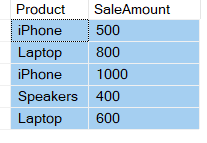Please use the following SQL Script to create and populate the Sales table with test data

```Create table Sales
(
Product nvarchar(50),
SaleAmount int
)
Go

Insert into Sales values ('iPhone', 500)
Insert into Sales values ('Laptop', 800)
Insert into Sales values ('iPhone', 1000)
Insert into Sales values ('Speakers', 400)
Insert into Sales values ('Laptop', 600)
Go
```
##### Example:

To calculate total sales by product, we would write a GROUP BY query as shown below

```SELECT Product, SUM(SaleAmount) AS TotalSales
FROM Sales
GROUP BY Product
```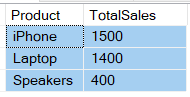Now if we want to find only those products where the total sales amount is greater than \$1000, we will use the HAVING clause to filter products as shown in the below SQL query

```SELECT Product, SUM(SaleAmount) AS TotalSales
FROM Sales
GROUP BY Product
HAVING SUM(SaleAmount) > 1000
```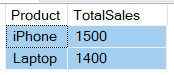If we use the Where clause instead of the Having clause, then we will get a syntax error. The reason is the Where clause in SQL Server will not work with the aggregate functions such as sum, min, max, avg, etc.

```SELECT Product, SUM(SaleAmount) AS TotalSales
FROM Sales
GROUP BY Product
WHERE SUM(SaleAmount) > 1000
```

When we execute the above query it will give us the error as Incorrect syntax near the keyword ‘WHERE’.

So, in short, the difference is the WHERE clause cannot be used with aggregate function whereas the HAVING clause can. The Where clause filters rows before aggregate calculations are performed where as HAVING clause filters rows after aggregate calculations are performed. Let us understand this with an example.

We can calculate the Total sales of iPhone and Speakers using either the WHERE clause or by using the HAVING clause. Calculate Total sales of iPhone and Speakers using WHERE clause: In the below example, the WHERE clause retrieves only the products such as iPhone and Speaker and then calculates the sum.

```SELECT Product, SUM(SaleAmount) AS TotalSales
FROM Sales
WHERE Product in ('iPhone', 'Speakers')
GROUP BY Product
```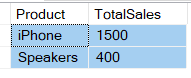Now we are going to Calculate the Total sales of iPhone and Speakers by using HAVING clause: The following example retrieves all the rows from the Sales table, and then only performs the sum operation. Once it performs the SUM operations then it removes all products from the result set except the iPhone and Speakers.

```SELECT Product, SUM(SaleAmount) AS TotalSales
FROM Sales
GROUP BY Product
HAVING Product in ('iPhone', 'Speakers')
```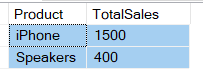So, from the performance point of view, the HAVING is slower than WHERE clause and the having clause should be avoided if possible.

##### Difference Between Where and Having Clause in SQL Server
1. WHERE clause cannot be used with aggregate functions whereas HAVING clause can be used with aggregate functions. This means WHERE clause is used for filtering individual rows on a table whereas HAVING clause is used to filter groups.
2. WHERE comes before GROUP BY. This means WHERE clause filters rows before aggregate calculations are performed. HAVING comes after GROUP BY. This means HAVING clause filters rows after aggregate calculations are performed. So, from a performance standpoint, HAVING is slower than WHERE and should be avoided when possible.
3. WHERE and HAVING clause can be used together in a SELECT query. In this case WHERE clause is applied first to filter individual rows. The rows are then grouped and aggregate calculations are performed, and then the HAVING clause filters the groups.
4. WHERE clause can be used with – Select, Insert, and Update statements whereas HAVING clause can only be used with the Select statement.

In the next article, I am going to discuss the Arithmetic Operators in SQL Server with examples. In this article, I try to explain the Difference Between Where and Having Clause in SQL Server with an example. I hope this article will help you with your need.